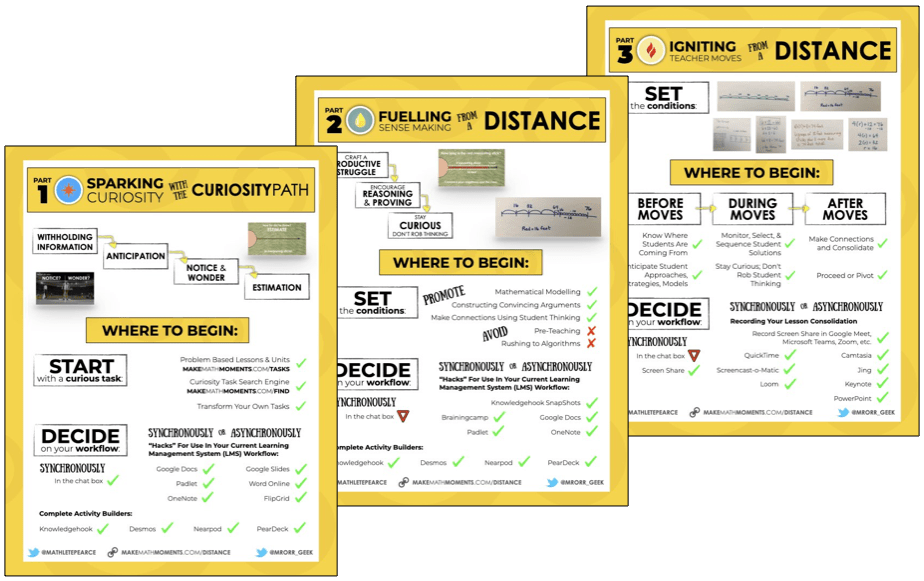In this video, we introduce the idea of subtracting integers by using simple subtraction of a positive by a positive (black squares) and negative subtracting a negative (red squares). We then offer a notice and wonder situation when we give black squares, followed by the introduction of a black and red (the zero principle).

Viewing the previous two posts would be useful for students to have the full understanding of the zero principle. Access them here and here.

Once students watch the introductory video above, show them this video:

Here, we try to do the opposite of what was happening in the previous two posts. Instead of combining a red and a black and having them disappear, we are having a red and black appear before subtracting one of the two colours.

Still not sure what is going on here? No problem! Check out the visual prompts below…

Use the following visual prompts to help students to conceptualize the addition and subtraction of integers.

Math is visual. Let’s teach it that way!

## Math Is Visual Prompts

Feel free to use the video and pause in-between ideas or alternatively, use the following visual prompts.

#### Visual #1 Prompts

Show the following image: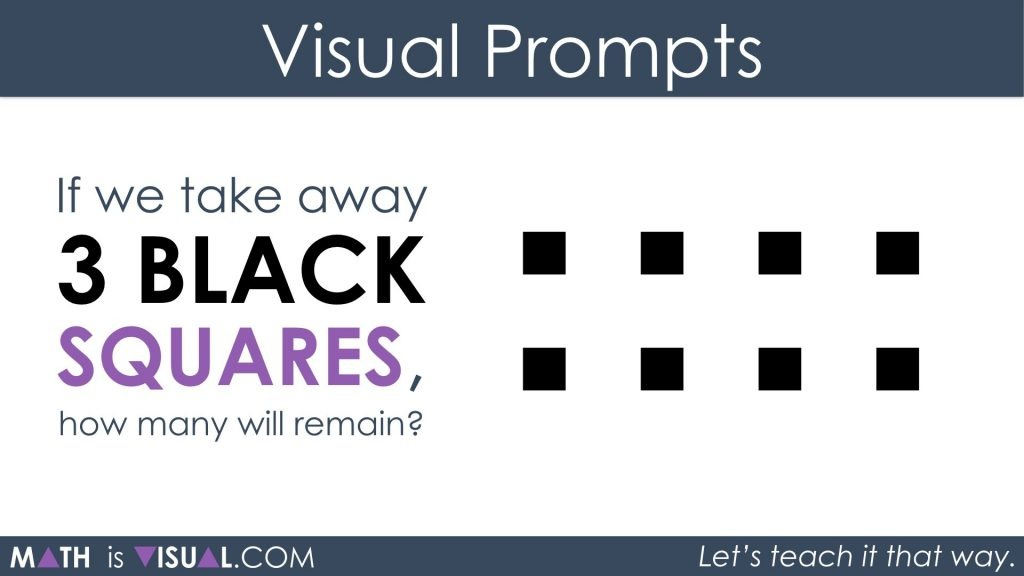You may consider working only with the visual prior to introducing the numerical symbols included in the visual below: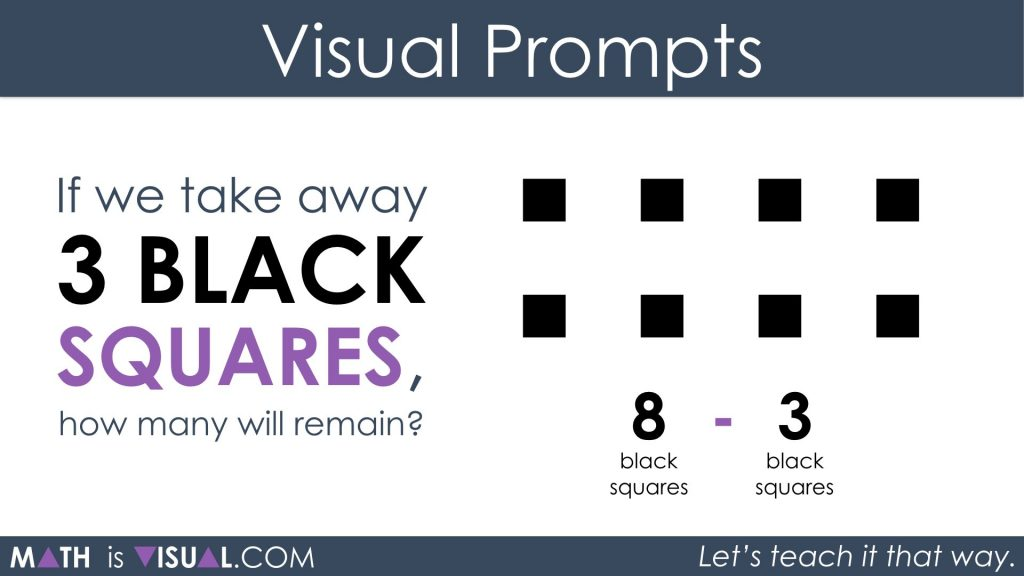After giving students some time to determine how many squares will remain and what colour they will be, you can show them the visual that matches this scenario.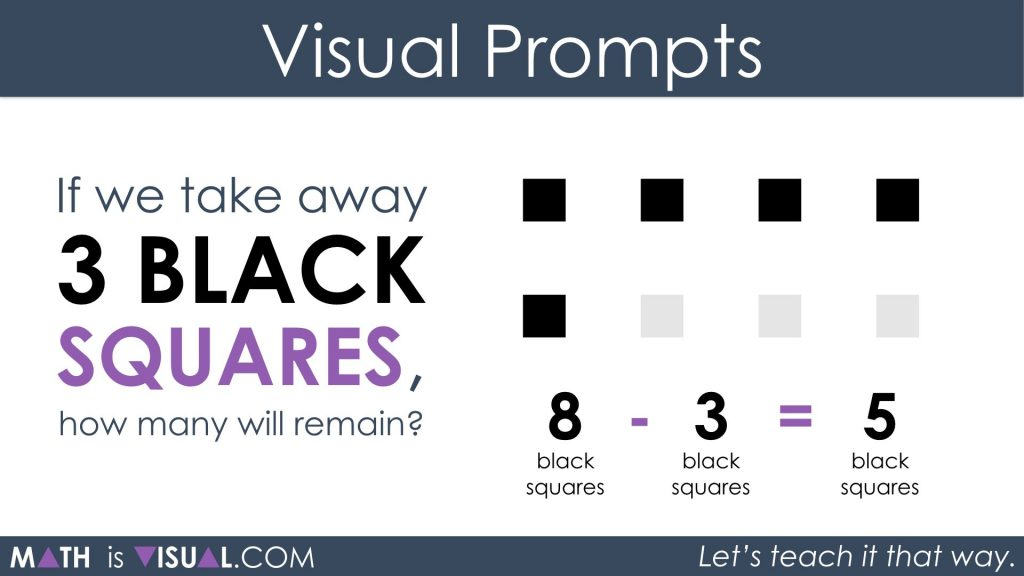#### Visual #2 Prompts

Show the following image: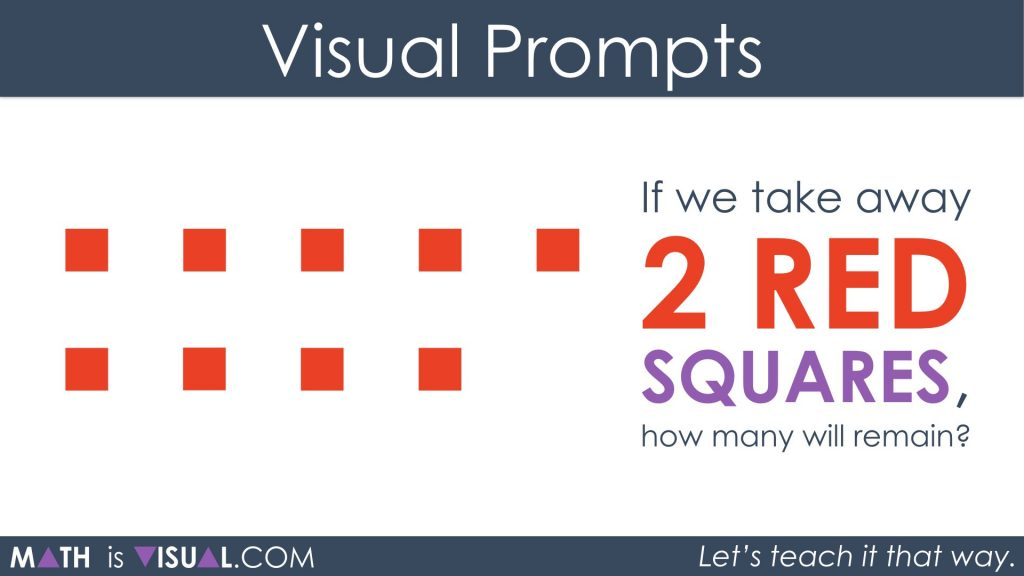After students use square tiles and come to their conclusion, have them write out their thinking using a numerical expression.

Then, show them this:And finally, a simplified version:#### Visual #3 Prompts

Show the following image:After students use square tiles and come to their conclusion, have them write out their thinking using a numerical expression.

Then, show them this: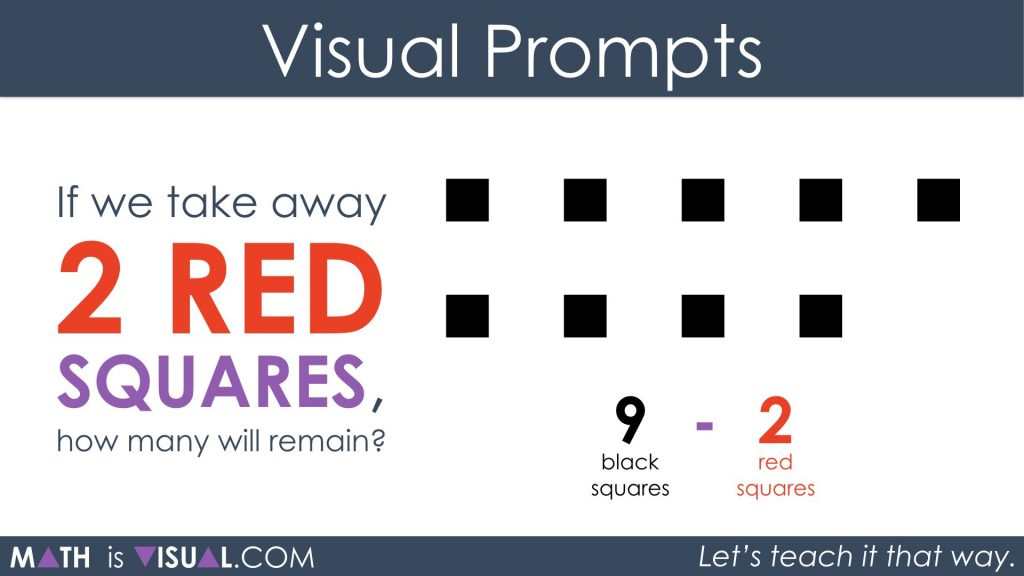And finally, visuals for the simplification: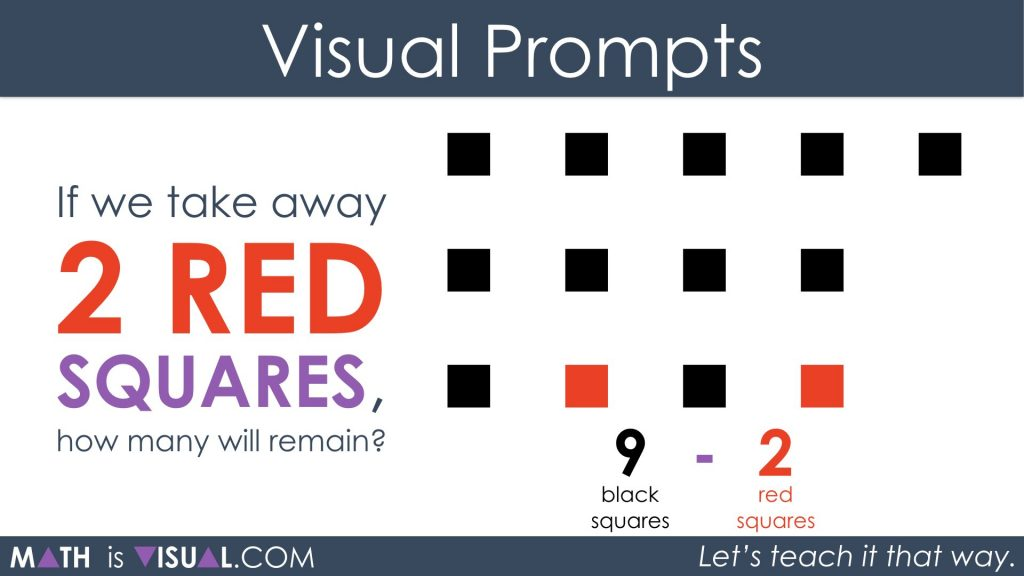#### Visual #4 Prompts

Show the following image: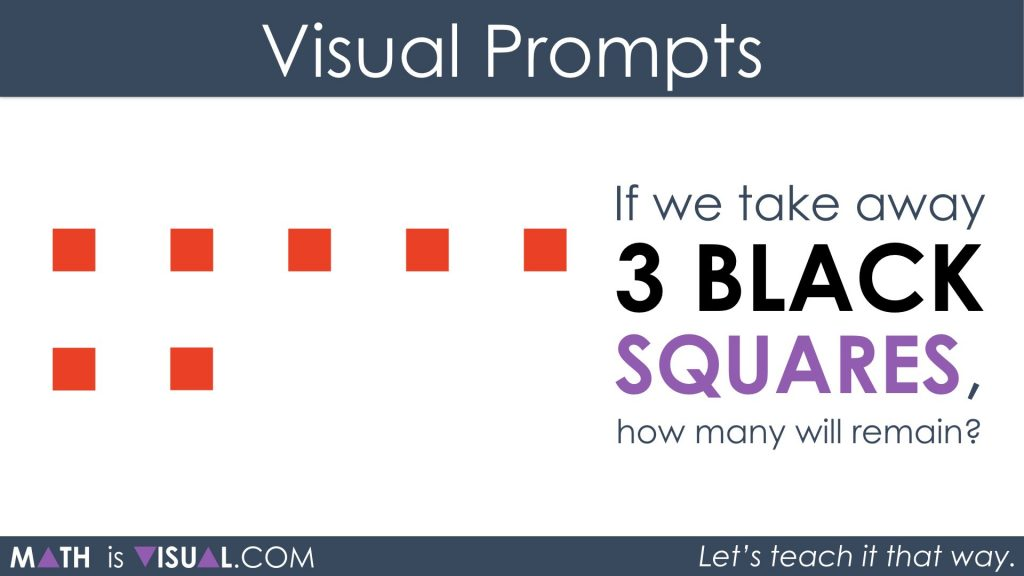After students use square tiles and come to their conclusion, have them write out their thinking using a numerical expression.

Then, show them this: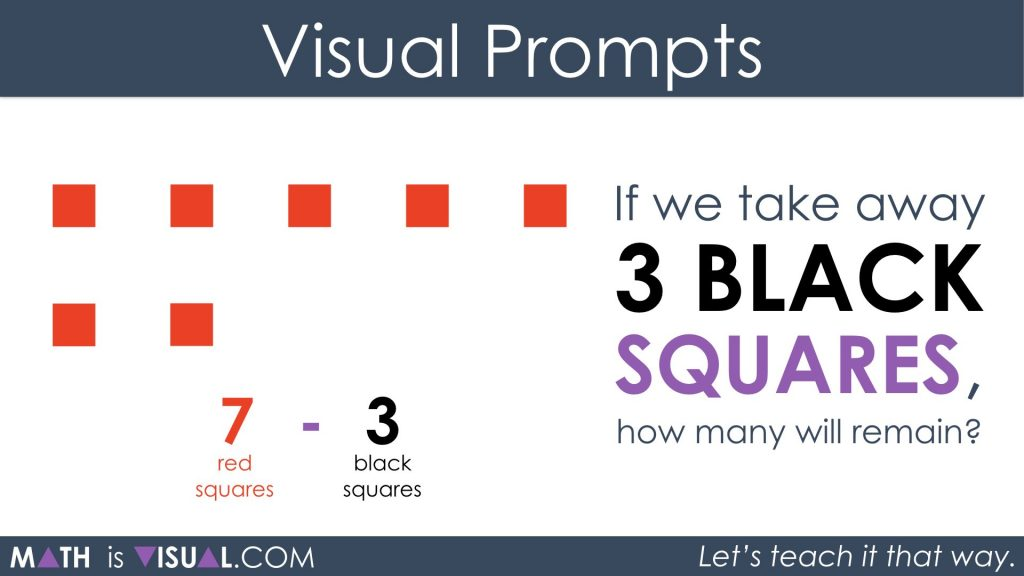And finally, a simplified version: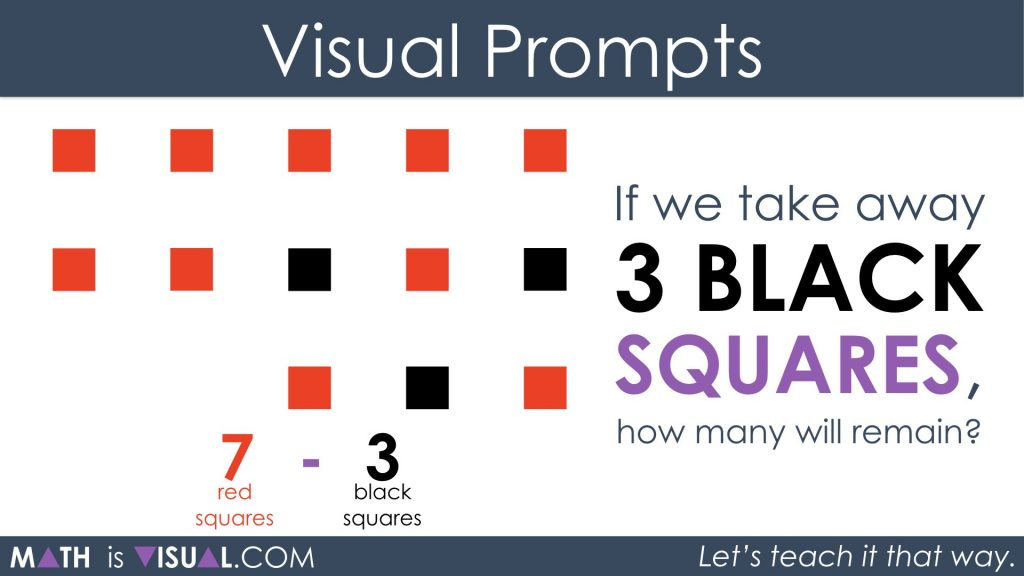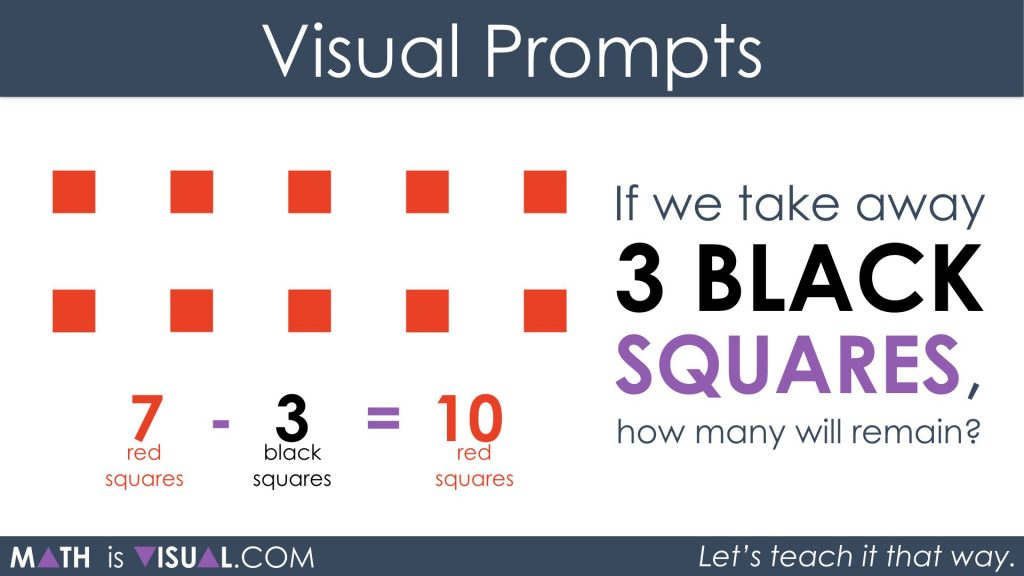#### kylepearce3